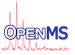OpenMS  2.8.0
Types.h File Reference
`#include <OpenMS/config.h>`
`#include <ctime>`
`#include <cstddef>`
`#include <limits>`
Include dependency graph for Types.h:

Go to the source code of this file.

## Namespaces

OpenMS
Main OpenMS namespace.

OpenMS::Internal
Namespace used to hide implementation details from users.

## Typedefs

typedef OPENMS_INT32_TYPE Int32
Signed integer type (32bit) More...

typedef OPENMS_UINT32_TYPE UInt32
Unsigned integer type (32bit) More...

typedef OPENMS_INT64_TYPE Int64
Signed integer type (64bit) More...

typedef OPENMS_UINT64_TYPE UInt64
Unsigned integer type (64bit) More...

typedef time_t Time
Time type. More...

typedef unsigned int UInt
Unsigned integer type. More...

typedef int Int
Signed integer type. More...

typedef OPENMS_BYTE_TYPE Byte
Byte type. More...

typedef OPENMS_UINT64_TYPE UID
A unique object ID (as unsigned 64bit type). More...

typedef size_t Size
Size type e.g. used as variable which can hold result of size() More...

typedef ptrdiff_t SignedSize
Signed Size type e.g. used as pointer difference. More...

## Enumerations

enum  ASCII {
ASCII__BACKSPACE = '\b' , ASCII__BELL = '\a' , ASCII__CARRIAGE_RETURN = '\r' , ASCII__HORIZONTAL_TAB = '\t' ,
ASCII__NEWLINE = '\n' , ASCII__RETURN = ASCII__NEWLINE , ASCII__SPACE = ' ' , ASCII__TAB = ASCII__HORIZONTAL_TAB ,
ASCII__VERTICAL_TAB = '\v' , ASCII__COLON = ':' , ASCII__COMMA = ',' , ASCII__EXCLAMATION_MARK = '!' ,
ASCII__POINT = '.' , ASCII__QUESTION_MARK = '?' , ASCII__SEMICOLON = ';'
}

## Functions

Numbers of digits used for writing floating point numbers (a.k.a. precision).

These functions are provided to unify the handling of this issue throughout OpenMS. (So please don't use ad-hoc numbers ;-) )

If you want to avoid side effects you can use precisionWrapper() to write a floating point number with appropriate precision; in this case the original state of the stream is automatically restored afterwards. See precisionWrapper() for details.

In practice, the number of decimal digits that the type can represent without loss of precision are 6 digits for single precision and 15 digits for double precision. We haveand, so rounding will remove the remaining difference.

Example:

#define NUMBER 12345.67890123456789012345678901
std::cout << NUMBER << '\n'; // default precision, writes: 12345.7
double d = NUMBER;
std::cout.precision(writtenDigits<double>(0.0)); // explicit template instantiation
std::cout << writtenDigits<double>(0.0) << ": " << d << '\n'; // writes: 15: 12345.6789012346
float r = NUMBER;
std::cout.precision(writtenDigits(r)); // type deduced from argument
std::cout << writtenDigits(r) << ": " << r << '\n'; // writes: 6: 12345.7
long double l = NUMBER;
std::cout.precision(writtenDigits(1L)); // argument is not used, but L suffix indicates a long double
std::cout << writtenDigits(1L) << ": " << l << '\n'; // writes: 18: 12345.6789012345671
double x = 88.99;
std::cout.precision(15);
std::cout << "15: " << x << '\n'; // writes: 15: 88.99
std::cout.precision(16);
std::cout << "16: " << x << '\n'; // writes: 16: 88.98999999999999
constexpr Int writtenDigits< double >(const double &)
Number of digits commonly used for writing a double (a.k.a. precision).
Definition: Types.h:219
constexpr Int writtenDigits(const FloatingPointType &=FloatingPointType())
Number of digits commonly used for writing a floating point type (a.k.a. precision)....
Definition: Types.h:294
template<typename FloatingPointType >
constexpr Int writtenDigits (const FloatingPointType &=FloatingPointType())
Number of digits commonly used for writing a floating point type (a.k.a. precision). Specializations are defined for float, double, long double. More...

template<>
constexpr Int writtenDigits< float > (const float &)
Number of digits commonly used for writing a `float` (a.k.a. precision). More...

template<>
constexpr Int writtenDigits< double > (const double &)
Number of digits commonly used for writing a `double` (a.k.a. precision). More...

template<>
constexpr Int writtenDigits< int > (const int &)
We do not want to bother people who unintentionally provide an int argument to this. More...

template<>
constexpr Int writtenDigits< unsigned int > (const unsigned int &)
We do not want to bother people who unintentionally provide an unsigned int argument to this. More...

template<>
constexpr Int writtenDigits< long int > (const long int &)
We do not want to bother people who unintentionally provide a long int argument to this. More...

template<>
constexpr Int writtenDigits< unsigned long int > (const unsigned long int &)
We do not want to bother people who unintentionally provide an unsigned long int argument to this. More...

template<>
constexpr Int writtenDigits< DataValue > (const DataValue &)
DataValue will be printed like double. More...

template<>
constexpr Int writtenDigits< long double > (const long double &)
Number of digits commonly used for writing a `long` `double` (a.k.a. precision). ... More...

## Variables

const char * OpenMS_locale